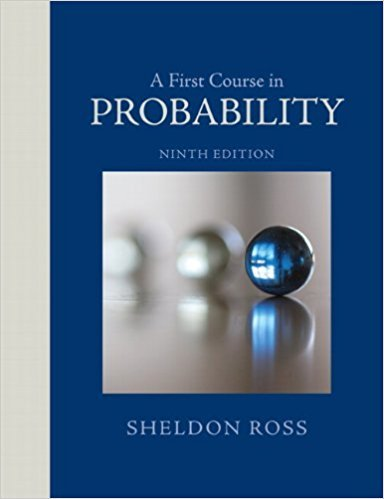×
Get Full Access to A First Course In Probability - 9 Edition - Chapter 4 - Problem 23p
Get Full Access to A First Course In Probability - 9 Edition - Chapter 4 - Problem 23p

×

# You have \$1000, and a certain commodity presently sellsISBN: 9780321794772 63

## Solution for problem 23P Chapter 4

A First Course in Probability | 9th Edition

• Textbook Solutions
• 2901 Step-by-step solutions solved by professors and subject experts
• Get 24/7 help from StudySoup virtual teaching assistantsA First Course in Probability | 9th Edition

4 5 1 325 Reviews
19
2
Problem 23P

Problem 23P

You have \$1000, and a certain commodity presently sells for \$2 per ounce. Suppose that after one week the commodity will sell for either \$ 1 or \$4 an ounce, with these two possibilities being equally likely.

(a) If your objective is to maximize the expected amount of money that you possess at the end of the week, what strategy should you employ?

(b) If your objective is to maximize the expected amount of the commodity that you possess at the end of the week, what strategy should you employ?

Step-by-Step Solution:

Solution:

Step 1 of 3:

We have \$1000, and a certain commodity presently sells for \$2 per ounce.

After one week the commodity will sell for either \$1 or \$4 an ounces, with theses two possibilities being equally likely.

1. We have to find strategy in order to maximize the expected amount of money.
2. We have to find strategy in order to maximize the expected amount of the commodity.

Step 2 of 3

Step 3 of 3

##### ISBN: 9780321794772

Unlock Textbook Solution